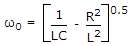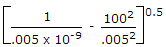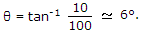Top

# Networks Analysis and Synthesis

1.

A two branch parallel tuned circuit has a coil of resistance 100 Ω and inductance 0.005 H in one branch and 10 nF capacitor in the second branch. At resonance frequency is== 44.67 x 104 rad/sec.

Enter details here

2.

A network has two branches in parallel. One branch contains impedance Z1 and the other branch has impedance Z2. If it is fed from an ac voltage V of frequency f, the current through Z1 depends, on

In parallel branches, the current through any branch depends only on voltage, impedance and frequency only.

Enter details here

3.

A capacitor is needed for an ac circuit of 230 V, 50 Hz the peak voltage rating of the capacitor should be

Peak voltage rating = 2 (rms voltage rating).

Enter details here

4.

In an RC series circuit R = 100 Ω and XC = 10 Ω. In this circuit

In RC circuit the current leads the voltage.Enter details here

5.

The current rating of a cable depends on

Current rating depends only on area of cross-section and hence diameter.

Enter details here

6.

Which of the following pairs are correctly matched?

1.Brune's realisation : realisation with ideal transformer.

3.Bott Duffin realisation : with non ideal transformer.

Select the answer using the following codes:

Bott Duffins realisation does not use transformer.

Enter details here

7.

The drift velocity of electrons is

Drift velocity of electrons is very small.

Enter details here

8.

A dielectric does not have leakage current.

All dielectrics have some leakage current.

Enter details here

9.

A capacitor stores 0.15C at 5 V. Its capacitance is

Q = CV or 0.15 = C(5) or C = 0.03 F.

Enter details here

10.

A 0.5 μF capacitor is connected across a 10 V battery. After a long time, the circuit current and voltage across capacitor will be